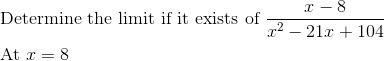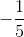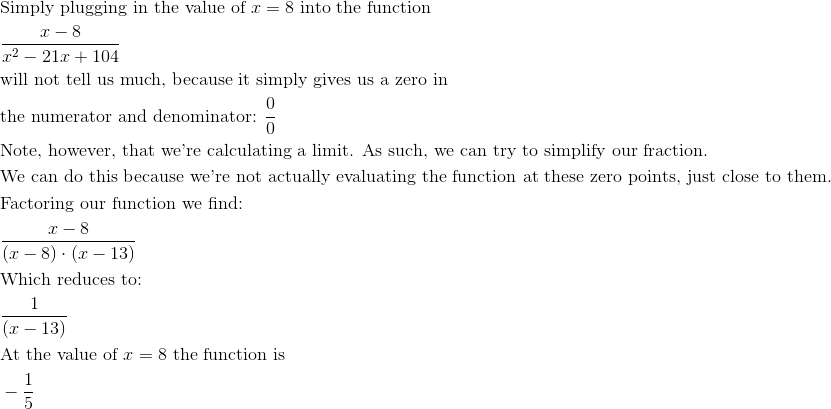AP Calculus AB : Calculating limits using algebra

Example Questions

← Previous 1 3 4

Example Question #1 : Calculating Limits Using Algebra

Calculate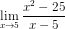5

100

The limit does not exist

10

Explanation:

First we notice that substituting 5 in for x will give us a 0 in the denominator.

So we simplify the equation by noticing the numerator is the difference of two squares.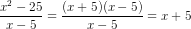Now we can substitute 5 in for x, and we arrive at our answer of 10.

Example Question #2 : Calculating Limits Using Algebra

Evaluate the following limit: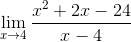Explanation:

Factor x-4 out of the numerator and simplify: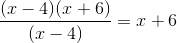Evaluate the limit for x=4: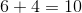Although there is a discontinuity at x=4, the limit at x=4 is 10 because the function approaches ten from the left and right side.

Example Question #3 : Calculating Limits Using Algebra

Evaluate the following limit: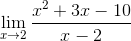Explanation:

Factor the numerator and simplify the expression.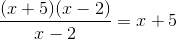Evaluate the function at x=2.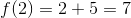There is a discontinuity at x=2, but since it the limit as x approaches 2 from the right is equal to the limit as x approaches 2 from the left, the limit exists.

Example Question #4 : Calculating Limits Using Algebra

Evaluate the following limit: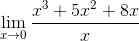does not existExplanation:

Factor the numerator to evaluate the limit: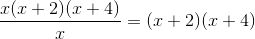Evaluate the limit: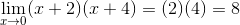There is a discontinuity at x=0 but the limit is equal to 8 because the limit from the right is equal to the limit from the left.

Example Question #5 : Calculating Limits Using Algebra

Evaluate the following limit: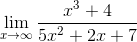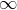Explanation:

As x approaches infinity, the x values that are raised to the highest power dominate the function and all other values become negligible. In this limit, x3 has the highest order in the numerator and 5x2 has the highest power of all the values in the denominator. We can simplify the limit to the following: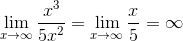After factoring out x2 it is easy to see that the limit diverges to infinity.

Example Question #1 : Limits Of Functions (Including One Sided Limits)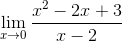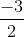Undefined.Explanation:

The first step to evaluating limits is always attempt to direct substitute the value into the function.  In this case, that is all you need to do.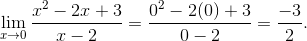Example Question #7 : Calculating Limits Using Algebra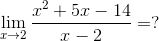Undefined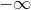Explanation:

To start this problem, we need to factor the quadratic equation.  This will result in a hole at, which will allow us to directly substitute the limit value into the resulting function to determine the limit.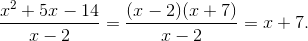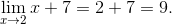Example Question #8 : Calculating Limits Using Algebra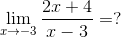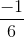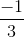Undefined.Explanation:

To evaluate this limit, we need to direct substitute the value in question into our function.  Note, this value is in the domain of the function, so direct substitution is the only method we need here.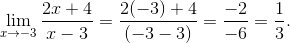Example Question #9 : Calculating Limits Using Algebra

Evaluate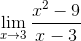Explanation:

This limit can't be evaluated by a simple substitution;is not defined at, so some simplification is in order first.. (Start)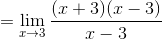. (Factor the numerator)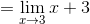. (Cancel the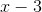terms)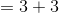. (Substitute in the)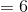.

Example Question #10 : Calculating Limits Using Algebra